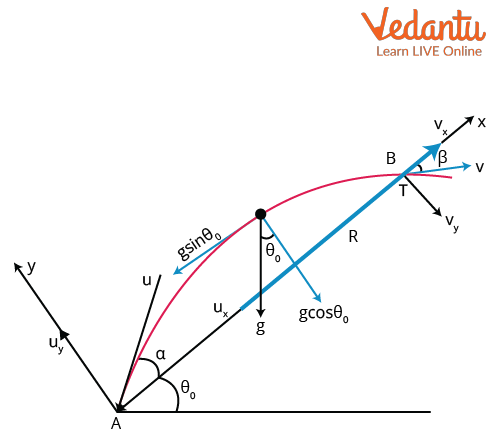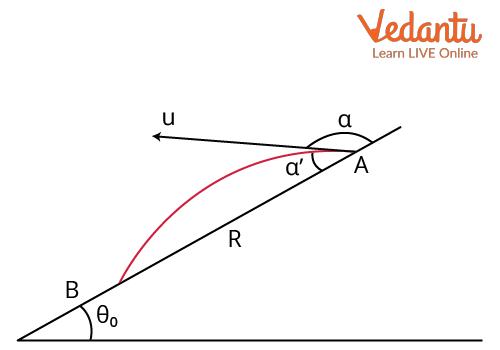Courses
Courses for Kids
Free study material
Free LIVE classes
More

# Projectile on Inclined Plane, Time of Flight and Trajectory for JEE## Introduction to Projectile Motion on Inclined Plane

Last updated date: 20th Mar 2023
Total views: 78.6k
Views today: 1.57k

In essence, the projectile motion of a body refers to the motion when an object is thrown into the air in the presence of the Earth's gravitational field. We could simply say that the motion of any object under the force of gravitation is known as projectile motion. There are many examples that we see in our daily lives, such as throwing a ball into the air, which shows projectile motion. There are some assumptions that have been made in the projectile motion, which are that the effects due to the rotation of the earth and resistance due to air are ignored in this motion. An object travelling in the shape of a parabola has both vertical and horizontal motion in the form of a projectile.

If the projectile motion is occurring on an inclined plane which is making some angle with the earth, then we call it projectile motion on an inclined plane. Let us look into projectile motion on inclined planes in detail, discussing how projectiles on an inclined plane travel and what the range of projectiles on inclined planes is.

## Range of Projectile on an Inclined Plane

Let us assume a ball is thrown upwards on an inclined plane, which makes an angle θ0 with the horizontal surface of the earth. The ball is thrown with the velocity u and it makes an angle ⍺ with the inclined plane. The ball, which is in a projectile motion, will have an initial velocity, acceleration, and final velocity. Each quantity can be divided into two components in the XY plane, as shown in the figure.Projectile on Inclined Plane

In the above figure, we can see that the path of the ball or projectile is from A to B.  The inclined surface makes an angle θ0 with the horizontal and the ball is thrown with the initial velocity u at an angle of ⍺ with the inclined surface.

The X-axis is taken parallel to the inclined plane and the Y-axis is perpendicular to the inclined plane, as shown in the figure. The horizontal and vertical components of initial velocity in the X and Y directions are ux and uy , respectively.

Here, u= ucos⍺ and uy = usin⍺

The acceleration on a projectile is the acceleration due to gravity and it is acting downwards in a negative XY axes direction. So, it has a negative sign. The components are divided as shown in the figure.

I.e., $a_{x}=-g \sin \theta_{0}$ and $a_{y}=-g \cos \theta_{0}$

The distance travelled by the projectile from A to B is in the x-direction, So Sx = R where R is the range and Sy = 0.

According to the Laws of motion, $\mathrm{S}=u t+\dfrac{1}{2} a t^{2}$

Let us take the equation of distance in the y-direction, i.e., Sy = 0

I.e., $S_{y}=u_{y} t+\dfrac{1}{2} a_{y} t^{2}$

Putting the values of uy and ay in the above equation,

\begin{align} &(u \sin \alpha) T-\dfrac{1}{2}\left(g \cos \theta_{o}\right) T^{2}=0 \\ &2(u \sin \alpha) T=\left(g \cos \theta_{o}\right) T^{2} \\ &T=\dfrac{2 u \sin \alpha}{g \cos \theta_{o}} \end{align}

This is the time of flight formula of a projectile on an inclined plane. Here, T is the total time taken to travel the distance from A to B and this is known as the time of flight.

Now, let us take the distance in the x-direction and substitute the x components in it.

\begin{align} &S_{x}=u_{x} t+\dfrac{1}{2} a_{x} t^{2} \\ &R=(u \cos \alpha) T-\dfrac{1}{2}\left(g \sin \theta_{o}\right) T^{2} \end{align}

Putting the value of T in this equation,

\begin{align} &R=(u \cos \alpha) \dfrac{2 u \sin \alpha}{g \cos \theta_{o}}-\dfrac{1}{2}\left(g \sin \theta_{o}\right)\left(\dfrac{2 u \sin \alpha}{g \cos \theta_{o}}\right)^{2} \\ &R=\dfrac{2 u^{2} \sin \alpha \cos \alpha}{g \cos \theta_{o}}-\dfrac{2 u^{2} \sin ^{2} \alpha \sin \theta_{o}}{g \cos ^{2} \theta_{o}} \end{align}

\begin{align}&R=\dfrac{u^{2} \sin 2 \alpha}{g \cos \theta_{o}}-\dfrac{2 u^{2} \sin ^{2} \alpha \sin \theta_{o}}{g \cos ^{2} \theta_{o}} \quad \text { As } 2 \sin \alpha \cos \alpha=\sin 2 \alpha \\ &R=\dfrac{u^{2}}{g \cos ^{2} \theta_{o}}\left[\left(\sin 2 \alpha \cos \theta_{0}\right)-\sin \theta_{0} 2 \sin ^{2} \alpha\right]\end{align}

We know, $2 \sin ^{2} \alpha=1-\cos 2 \alpha$

\begin{align} &R=\dfrac{u^{2}}{g \cos ^{2} \theta_{0}}\left[\left(\sin 2 \alpha \cos \theta_{0}\right)-\sin \theta_{0}(1-\cos 2 \alpha)\right] \\ &R=\dfrac{u^{2}}{g \cos ^{2} \theta_{0}}\left[\sin 2 \alpha \cos \theta_{0}-\sin \theta_{0}+\sin \theta_{0} \cos 2 \alpha\right]\end{align}

$\text {Therefore } \sin 2 \alpha \cos \theta_{0}+\sin \theta_{0} \cos 2 \alpha=\sin \left(2 \alpha+\theta_{0}\right)$

$R=\dfrac{u^{2}}{g \cos ^{2} \theta_{0}}\left[\sin \left(2 \alpha+\theta_{0}\right)-\sin \theta_{0}\right]$

Therefore, the equation of the range of projectile formula on the inclined plane is given by

$R=\dfrac{u^{2}}{g \cos ^{2} \theta_{0}}\left[\sin \left(2 \alpha+\theta_{0}\right)-\sin \theta_{0}\right]$

If the projectile is projected in the downward direction of the inclined plane, then the distance is taken in the negative x direction, i.e., - R and the angle is taken as | , as shown in the figure.Projectile down the inclined plane

Here $\alpha^{\prime}+\alpha=180$, so $\alpha=180-\alpha^{\prime}$

\begin{align} &-R=\dfrac{u^{2}}{g \cos ^{2} \theta_{o}}\left[\sin \left(2\left(180-\alpha^{\prime}\right)+\theta_{0}\right)-\sin \theta_{0}\right] \\ &-R=\dfrac{u^{2}}{g \cos ^{2} \theta_{o}}\left[\sin \left\{360-\left(2 \alpha^{\prime}-\theta_{0}\right)\right\}-\sin \theta_{0}\right]\end{align}

\begin{align} & -R=\dfrac{u^{2}}{g \cos ^{2} \theta_{o}}\left[-\sin \left(2 \alpha^{1}-\theta_{0}\right)-\sin \theta_{0}\right] \\ & R=\dfrac{u^{2}}{g \cos ^{2} \theta_{o}}\left[\sin \left(2 \alpha^{\prime}-\theta_{0}\right)+\sin \theta_{0}\right]\end{align}

This is the range of projectiles down the inclined plane formula. Let us look into the equation of the trajectory of the projectile.

## Equation of Trajectory of Projectile

Let us assume a general projectile on a horizontal plane is thrown with the velocity of u at an angle $\alpha$ with the horizontal surface, and the acceleration is the acceleration due to gravity, which acts along only the y-direction.

The horizontal distance, $x = (u\cos{\alpha})t$ as there is no acceleration due to gravity in the horizontal direction.

I.e.,$\mathrm{t}=\dfrac{x}{u \cos \alpha}$

The vertical distance,

$y=u \sin \alpha . t-\dfrac{1}{2} g t^{2}$

\begin{align} &\text { Where } t=\dfrac{x}{u \cos \alpha}\\ & y=(u \sin \alpha) \dfrac{x}{u \cos \alpha}-\dfrac{1}{2} g\left(\dfrac{x}{u \cos \alpha}\right)^{2}\\ &y=x \tan \alpha-\dfrac{g x^{2}}{2 u^{2} \cos ^{2} \alpha}\\ &y=x \tan \alpha-\dfrac{x^{2}}{\frac{2 u^{2} \cos ^{2} \alpha}{g}}\end{align}

\begin{align} &y=x \tan \alpha-\dfrac{x^{2}}{\dfrac{2 u^{2} \cos \alpha \sin \alpha}{g}} \dfrac{\sin \alpha}{\cos \alpha}\\ &y=x \tan \alpha-\dfrac{x^{2}}{\dfrac{u^{2} \sin 2 \alpha}{g}} \tan \alpha\\ &\text {Let } \dfrac{u^{2} \sin 2 \alpha}{g}=R\\ &y=x \tan \alpha\left(1-\dfrac{x}{R}\right) \end{align}

This is the equation for the trajectory of a projectile.

Let us solve a problem on the concept of projectile motion using the inclined plane formula.

Example: A ball is thrown from the foot of an inclined plane in an upward direction with a velocity of 10$\dfrac{m}{s}$ with an angle of 30 degrees with the inclined surface. If the inclined surface is making an angle of 30 degrees with the horizontal, find the range of the ball on the inclined plane.

Solution:

Given that, the initial velocity of the ball, u = 10$\dfrac{m}{s}$

The angle between the inclined surface and the horizontal is $\theta_0$ = 30o

The initial velocity makes an angle, $\alpha$ = 30o

We know the formula of the range travelled by the ball on a plane in an inclined position,

$R=\dfrac{u^{2}}{g \cos ^{2} \theta_{0}}\left[\sin \left(2 \alpha+\theta_{0}\right)-\sin \theta_{0}\right]$

Putting all the values given in the equation,

\begin{align}&R=\dfrac{10^{2}}{10 \cos ^{2} 30}[\sin (2(30)+30)-\sin 30] \\ &R=\dfrac{10 \times 4}{3}\left[1-\dfrac{1}{2}\right] \\&R=\dfrac{40}{3} \cdot \dfrac{1}{2} \\ &R=\dfrac{20}{3}=6.67 \mathrm{~m} \end{align}

Therefore, the distance travelled by the ball on the inclined plane is 6.67 metres.

## Conclusion

Projectile motion is a concept which studies the motion of objects in the presence of the gravitational field. Therefore, we learned about the range of projectile formulas on an inclined plane through this article. We also learnt how to derive the equation for the trajectory of a projectile and also the time of flight formula on the plane in an inclined position through this article.

## FAQs on Projectile on Inclined Plane, Time of Flight and Trajectory for JEE

1. What are the assumptions taken in projectile motion?

There are many assumptions which must be made when an object is in a projectile motion, either on a horizontal plane or an inclined plane. The resistance due to air is taken as zero on the projectile. The effects due to the curvature and rotation of the earth on the projectile are ignored in the projectile motion. The acceleration of gravity is taken as a constant both in magnitude and direction in projectile motion. These are the assumptions that are made in projectile motion.

2. What is the weightage of this topic in JEE?

The topic of the projectile in kinematics is very important in the JEE examination. There is a weightage of 3.33% and at least one question will be asked on this topic. There are some questions like finding the time of flight when two inclined planes 1 and 2 have an inclination of alpha beta and finding the range on the inclined plane for the projectile, which are important questions that are being asked in the JEE examination. So, learning the projectile motion and the motion of the projectile on an inclined plane is very important.